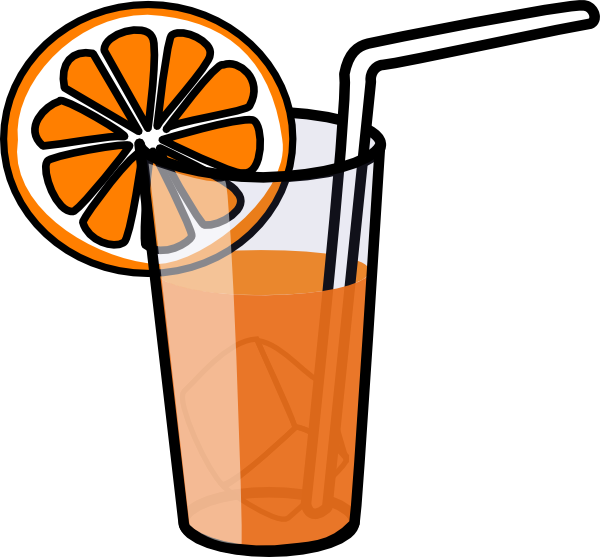# Clockwork Orange JuiceWe all love to have chilled drinks in summers. So here's a nice problem related to orange juice.

A jug contains 15 glasses of Orange Juice. When you open the tap at the bottom it takes 12 seconds to fill a glass with juice. If you leave the tap open, how long will it take to fill the remaining 14 glasses and thus empty the jug. This time can be expressed as $\frac { a\sqrt { b } }{ \sqrt { c } -\sqrt { d } }$, then find $a+b+c+d$.

Details and Assumptions:

• Consider the jug to be a cylinder.

• all 15 glasses are indistinguishable.

• $b, c$ and $d$ are square free positive integers.

×